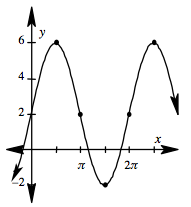### Home > PC > Chapter 6 > Lesson 6.2.3 > Problem6-94

6-94.
1. Use the graph shown at right to write possible equations. Homework Help ✎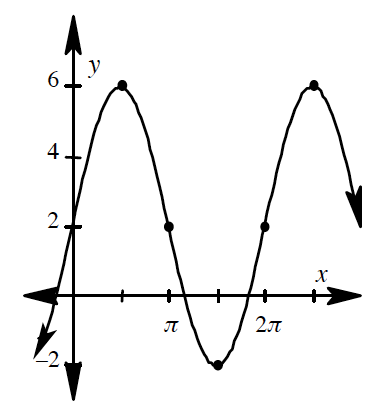1. Write an equation that is a transformation of sine.

2. Write an equation that is a transformation of cosine.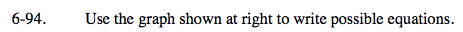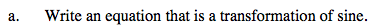Find the:
1. Amplitude
2. Vertical Shift
3. Frequency
4. Horizontal Shift.

Substitute the information in:
y = a sin(b(θ ± h)) ± k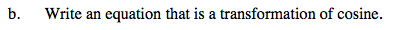Find the same information above. Substitute in:
y = a sin(b(θ ± h)) ± k